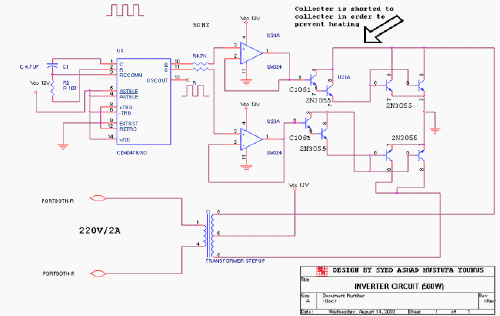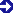# 500W low cost 12V to 220V inverter Electronic Schematics > Power > 500W low cost 12V to 220V inverter

Using this circuit you can convert the 12V dc in to the 220V Ac. In this circuit 4047 is use to generate the square wave of 50hz and  amplify the current and then amplify the voltage by using the step transformer.

Attention: This Circuit is using high voltage that is lethal. Please take appropriate precautionsModified Schematic - click on image for higher resolutionOriginal Schematic - click on image for higher resolution

 How to calculate transformer rating The basic formula is P=VI and between input output of the transformer we have Power input = Power output For example if we want a 220W output at 220V then we need 1A at the output. Then at the input we must have at least 18.3V at 12V because: 12V*18.3 = 220v*1 So you have to wind the step up transformer 12v to 220v but input winding must be capable to bear 20A.Download this schematic in Orcad format

Title: 500W low cost 12V to 220V inverter
electronic circuit
Source: www.electronics-lab.com
Published on: 2005-02-03
Print version: